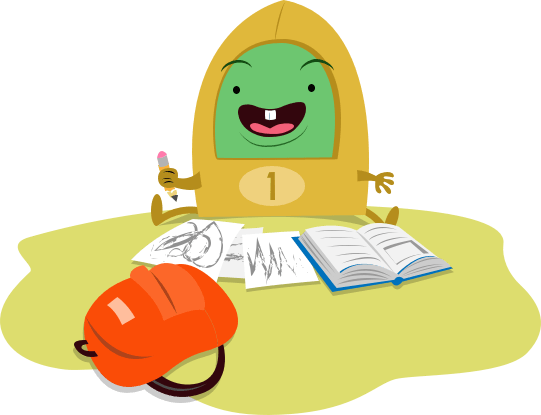# This Week in Seventh Grade

Week 39## Probability and Spelling

Learners will make predictions using experimental probability and theoretical probability in the context of real-world events. Then, they'll practice correct spelling and word usage, focusing on plurals, possessives, and commonly misspelled words.
Students will make predictions using experimental probability and theoretical probability in the context of real-world events. Then, they'll practice correct spelling and word usage, focusing on plurals, possessives, and commonly misspelled words.
Make Predictions Using Experimental Probability
Worksheet
Make Predictions Using Experimental Probability
In this seventh-grade probability worksheet, students are introduced to the concept of experimental probability and shown how to use this information to make predictions.
Math
Worksheet
Make Predictions Using Theoretical Probability
Worksheet
Make Predictions Using Theoretical Probability
In this seventh-grade probability worksheet, students will practice finding the theoretical probabilities for different events and using those to make predictions.
Math
Worksheet
Spelling With Plurals and Possessives
Worksheet
Spelling With Plurals and Possessives
Practice spelling with plurals and possessives in this grammar worksheet for middle grades!
Worksheet
Correct Spelling Errors
Worksheet
Correct Spelling Errors
Give students practice identifying and correcting misused and misspelled words in context with this helpful grammar worksheet!
WorksheetSend home these fun free resources to help reinforce the skills you're teaching in the classroom.